# Bollinger Bands

Bollinger Bands are a kind of trading envelope. Bollinger Bands consist of a moving average and two standard deviations charted as one line above and one line below the moving average. The line above is two standard deviations added to the moving average. The line below is two standard deviations subtracted from the moving average.

Traders generally use them to determine overbought and oversold zones, to confirm divergences between prices and indicators, and to project price targets. The wider the bands are, the greater the volatility is. The narrower the bands are, the lesser the volatility is. The moving average is calculated on the close.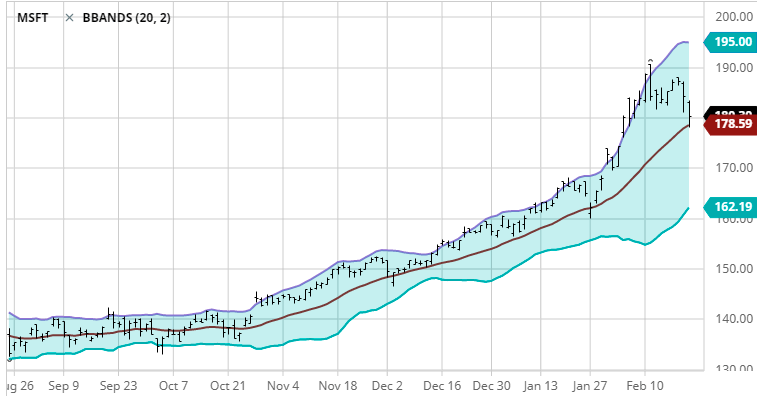Parameters:

• Period (20) – the number of bars, or period, used to calculate the study. John Bollinger, the creator of this study, states that those periods of less than ten days do not seem to work well for Bollinger Bands®. He says that the optimal period for most applications is 20 or 21 days.
• Band Width (2) – The half-width of the band in terms multiples of standard deviation. Typically 2 is used. John Bollinger suggests, if you reduce the number of days used to calculate the bands, you should also reduce the number of deviations and vise versa. For example, if you use a period of 50, you may want to use 2.5 as the standard deviation. For a period of 10, you may want to use 1.5 or 1.0. The application uses a default of 2.0
• Shift (0) – the number of bars to displace the moving average.

Computation

1. Calculate the moving average. The formula is: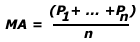• Pn the price you pay for the nth interval
• n the number of periods you select
2. Subtract the moving average from each of the individual data points used in the moving average calculation. This gives you a list of deviations from the average. Square each deviation and add them all together. Divide this sum by the number of periods you selected.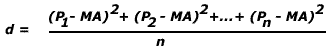1. Take the square root of d. This gives you the standard deviation.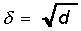1. Compute the bands by using the following formulas: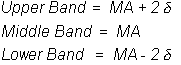• Pn is the price you pay for the nth interval
• n is the number of periods you select

Interpretation

Traders generally use Bollinger Bands® to determine overbought and oversold zones, to confirm divergences between prices and studies, and to project price targets. The wider the bands, the greater the volatility. The narrower the bands, the lesser the volatility.

Some authors recommend using Bollinger Bands in conjunction with another study, such as the RSI. If price touches the upper band and the study does not confirm the upward move (i.e. there is divergence), a sell signal is generated. If the study confirms the upward move, no sell signal is generated, and in fact, a buy signal may be indicated. If price touches the lower band and the study does not confirm the downward move, a buy signal is generated. If the study confirms the downward move, no buy signal is generated, and in fact, a sell signal may be indicated.

Another strategy uses the Bollinger Bands alone. In this approach, a chart top occurring above the upper band followed by a top below the upper band generates a sell signal. Likewise, a chart bottom occurring below the lower band followed by a bottom above the lower band generates a buy signal.

Bollinger Bands also help determine overbought and oversold markets. When prices move closer to the upper band, the market is becoming overbought, and as the prices move closer to the lower band, the market is becoming oversold. The market’s price momentum should also be taken into account. When a market enters an overbought or oversold area, it may become even more so before it reverses. You should always look for evidence of price weakening or strengthening before anticipating a market reversal.

Bollinger Bands can be applied to any type of chart, although this study works best with daily and weekly charts. When applied to a weekly chart, the Bands carry more significance for long-term market changes.